# Moderated regression analysis

### Introduction

Moderator models are often used to examine when an independent variable influences a dependent variable. That is, moderated models are used to identify factors that change the relationship between independent and dependent variables.

To illustrate, suppose you wanted to determine whether or not the impact of watching Big Brother and Temptation Island on intelligence depends on your memory span. That is, only individuals who can remember many objects at the same time can learn from the subtle insights portrayed in Big Brother and Temptation Island. An extract of the data is presented below. Each row corresponds to one participant.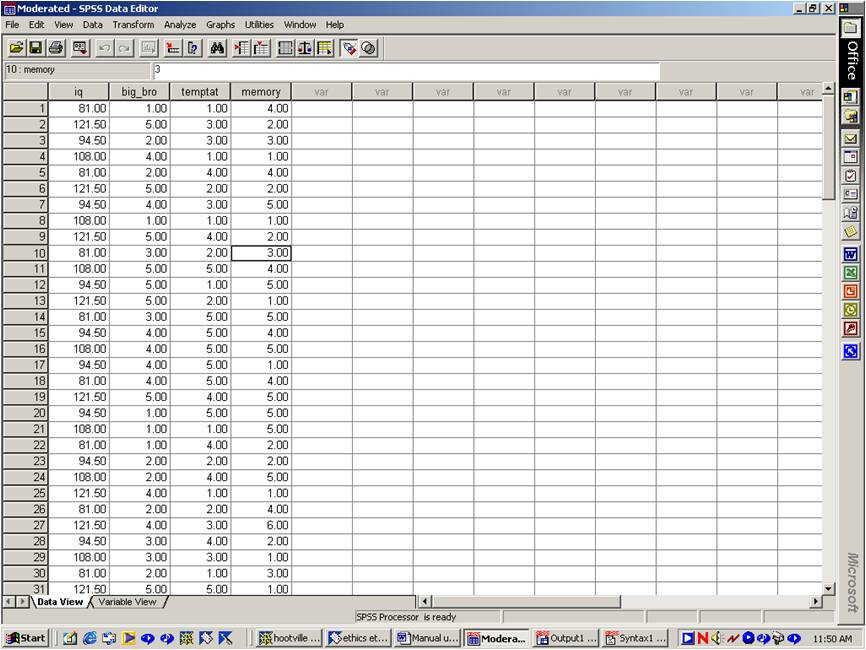To resolve this question, we need to determine whether or not the effect of Big Brother on IQ depends on memory span. In addition, we need to determine whether or not the effect of Temptation Island on IQ depends on memory span. In other words, we need to ascertain whether or not memory span moderates or interacts with Big Brother and Temptation Island to influence IQ. Of course, we could:

• Categorize the variables Big Brother, Temptation Island, and memory span into groups, such as high, medium, and low.
• Once these variables are categorised, we could undertake ANOVAs to resolve our question.
• Unfortunately, this categorisation clearly restricts power, because subtle variations in our data are overlooked.
• Instead, we should explore this issue within the context of regression.
• That is, we should complete a variant of regression called moderated regression analysis.

### Step 1: Create the product terms

To examine interactions within the context of moderated regression, we need to create additional columns in our database. The first column will equal:

(Big Brother - mean of Big Brother) x (memory span - mean of memory span).

The second column will equal:

(Temptation Is - mean of Temptation Is) x (memory span - mean of memory span).

Hence, these columns essentially equal the product of each term, after the variables have been centered, a concept that will be clarified later. The first product term actually represents the interaction between Big Brother and memory span. The second product term actually represents the interaction between Temptation Is and memory span. These terms, which appear in the datasheet below, will be justified later. Your analysis might comprise one, two, three, or even more of these interactions terms.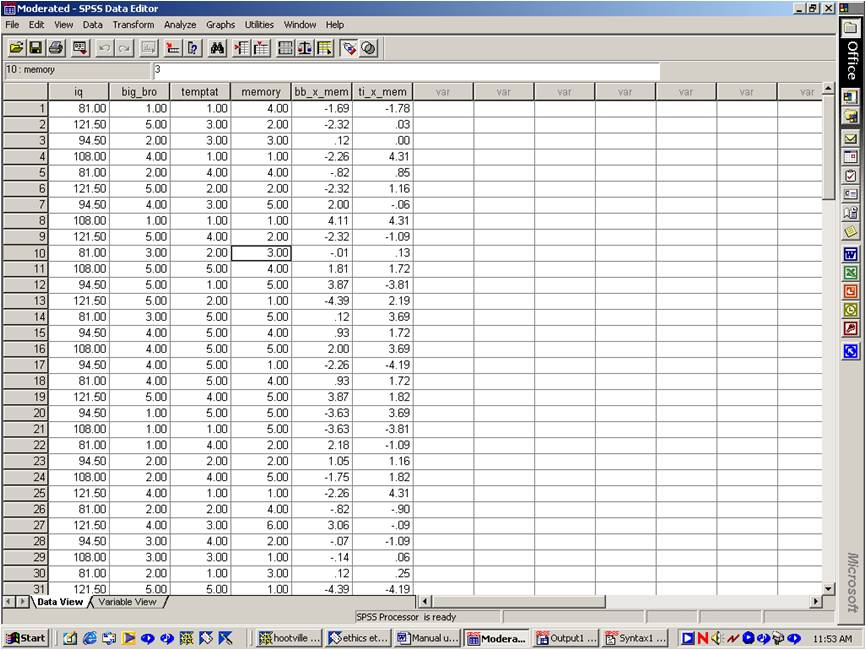To create these product terms, you first need to determine the mean of each variable. For instance, you could:

1. Select "Descriptives" and then "Descriptives" from the "Analyse Data" menu.
2. Transfer the labels that pertain to Big Brother, Temptation Island, and memory span into the box labelled "Variables". Press "OK" to generate a table of means.
3. Select "Compute" from the "Transform" menu.
4. In the box labelled "Target variable", enter a name to represent the new column or product term, such as "BB_x_Mem".
5. In the box labelled "Numerical Expression", specify the appropriate formula, such as (bigbro - 2.913) * (memory - 3.125).

In this example, 2.913 is the hypothetical mean score of Big Brother and 3.125 is the hypothetical mean score of memory span. Repeat this process to create the product term that represents the interaction between Temptation Island and memory span.

### Step 2: Undertake regression analysis

The next step is to complete the regression analysis. That is:

1. Select "Regression" and then "Linear" from the "Analyse Data" menu.
2. Designate IQ as the dependent variable.
3. Designate the product terms, as well as all the variables that were utilised to create these interactions, as the independent variables.
4. An extract of the output is presented below.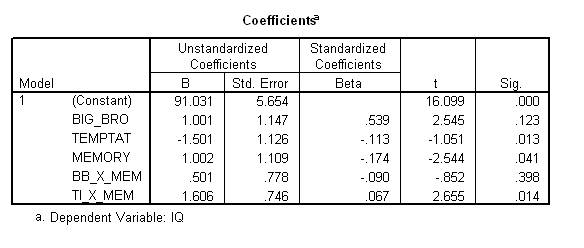### Step 3: Interpretation of significance

To interpret this output, you should examine the column labelled "sig", which represents the significance or p values that pertain to each independent variable. In this instance, the p value that pertains to the BB_x_mem term exceeds 0.05 and thus does not reach significance. Accordingly, the researcher would conclude that no evidence exists that memory span moderates or influences the impact of viewing Big Brother on intelligence. On the other hand:

• The p value that pertains to TI_x_mem is less than 0.05 and thus achieved significance.
• Hence, memory span moderates the effect of viewing Temptation Island on intelligence.

The other terms, called main effects, can also be interpreted. Specifically, the p value associated with Temptation Island is less than 0.05 and is thus significant. This finding suggests that viewing Temptation Island reduces intelligence. However, as we discovered earlier, memory span moderates this effect. In particular, this main effect applies when memory span is average. In other words, Temptation Island reduces intelligence when memory span is average. The impact of Temptation Island on intelligence at other levels of memory span will be explored below.

### Step 4: Interpretation of direction

To reiterate, the effect of Temptation Island on intelligence is influenced by memory span. The nature of this relationship, however, has not been explored. For instance:

• Temptation Island may reduce intelligence to a greater extent when memory span is elevated.
• On the other hand, Temptation Island may reduce intelligence to a greater extent when memory span is reduced.
• Several procedures can be conducted to distinguish these alternatives.

Perhaps the most informative procedure involves creating a graph. An example is presented below.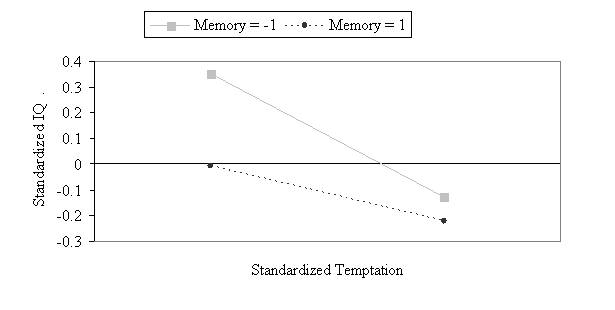To create this graph, the researcher should formulate equations that represent the relationship between Temptation Island and intelligence at various levels of memory span. The standardised Beta values, which appeared in the third column of the output, are utilised to generate these equations. Specifically, to generate these equations, you should:

• First utilise the standardised Beta values to represent the dependent variable--intelligence--as a function of all the other variables.
• In particular, each standardised Beta value represents the coefficient of each term in this equation.

In this instance, the equation equals:

Standardised values of IQ = 0.539 x BB - 0.113 x TI - 0.174 x Mem + 0.090 x BB_x_Mem + 0.067 x TI_x_Mem

You might not understand this step initially, but you should be able to follow the example anyway. Second, variables that are not relevant to the interaction of interest should be set to zero. The justification of this step is complex and assumes a comprehensive understanding of regression. To demonstrate, recall the researcher needs to determine the relationship between TI and IQ at various levels of Mem. Presumably, the researcher should explore these relationships at the average level of other variables, such as BB. The Standardised Beta values, however, represent the Beta values that would have been generated had the variables been converted to z scores. The mean of z scores is zero. Thus, to represent the average level of BB, this variable should be set to zero.

To reiterate, variables that are not relevant to the interaction of interest should be set to zero. For instance:

• If the researcher is interested in the interaction between TI and mem, the variable BB is not relevant and should be set to zero.
• Furthermore, all the terms that comprise BB should be set to zero.

The justification of the last recommendation is also very complex. To illustrate, BB_x_mem represents (BB - mean of BB) * (Mem - mean of MM). Because the means equal zero once the variables have been converted to z scores, BB_x_mem reduces to BB x Mem. Hence, when BB is set to zero, interaction terms that comprise BB also equal zero. Accordingly, the previous equation thus becomes:

Standardised values of IQ = -0.113 x TI - 0.174 x Mem + 0.067 x TI_x_Mem Eq (1)

Third, you need to estimate the dependent variable when both the independent variable and moderator are greater than average. In this instance, you could estimate IQ when both TI and Mem equal 1. Remember that all variables have been converted to z scores, and thus a value of 1 exceeds average. When TI and Mem are converted to 1, Equation (1) becomes:

Standardised values of IQ = -0.113 x 1 - 0.174 x 1 + 0.067 x 1 x 1 = -.22

Fourth, repeat this procedure, except estimate the dependent variable when:

• The independent variable equals -1 and the moderator equals 1
• The moderator equals 1 and the independent variable equals -1
• Both the independent variable and the moderator equals -1

For example, when TI is -1 and Mem is 1, Equation (1) becomes:

Standardised values of IQ = -0.113 x -1 - 0.174 x 1 + 0.067 x -1 x 1 = -.128

Likewise, when TI is 1 and Mem is -1, Equation (1) becomes:

Standardised values of IQ = -0.113 x 1 - 0.174 x -1 + 0.067 x 1 x -1 = .22

Furthermore, when TI is -1 and Mem is -1, Equation (1) becomes:

Standardised values of IQ = -0.113 x -1 - 0.174 x -1 + 0.067 x -1 x -1 = .354

Finally, to represent these equations in a graph:

• Open Microsoft Excel, as shown below.
• Enter words and numbers that resemble the example below.
• However, instead of "Memory" and "Temptation Island", specify the name of your moderator and independent variable.
• In addition, instead of the numbers -.22, .-.128, .22, and .354, specify the numbers that you calculated.
• For example, in cell B2, specify the number you calculated when the independent variable is -1 and the moderator is -1.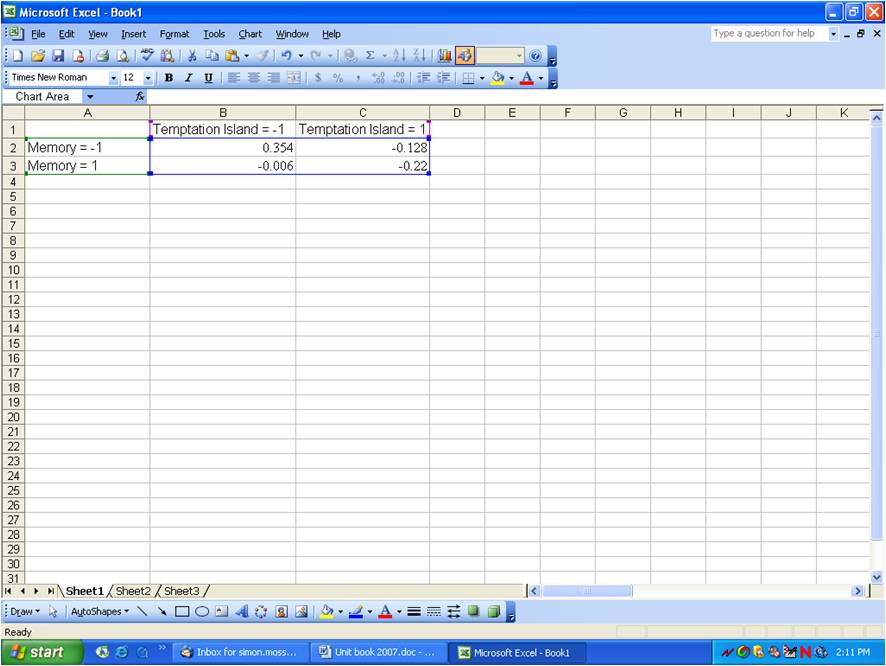To create the graph:

• Highlight all the cells from A1 to C3.
• Press the graph icon, which appears above column C in this example.
• Select "Line" and the chart type.
• Press Next twice.
• Label the Y axis, such as "Standardized IQ"&
• Label the X axis, such as "Standardized Temptation Island".
• Press "Next" and then "Finish"
• If you like, you can reformat the graph, which should resemble the example below.### Illustration of the format used to report moderated regression.

Multiple regression analysis was conducted to ascertain whether or not the relationship between watching Big Brother or Temptation Island and IQ depends on memory span. To represent the interaction between Big Brother or Temptation Island and memory span, the variables were first centered and then multiplied together. This analysis revealed the effect of Temptation Island, but not Big Brother, on IQ depends on working memory span. The standardised B and t values that emerged from this analysis are presented in Table 1.

Standardized B t
Constant17.0***
Big Brother .54 2.54
Temptation Island -.11 -1.05*
Memory Span -.17 -2.54*
Big Brother x Memory Span -.09 -.85
Temptation Island x Memory Spa .07 2.65*

* p < 0.05, ** p < 0.01, *** p < 0.001

Figure 1 presents the relationship between Temptation Island and IQ at high (z=1) and low (z=-1) levels of memory span. These equations were derived from the standardised B values. According to this figure, when memory span is limited, Temptation Island is inversely related to IQ. This relationship, however, diminishes as memory span increases.